# Introduction to JPEG Compression

In our last tutorial of image compression, we discuss some of the techniques used for compression

We are going to discuss JPEG compression which is lossy compression, as some data is loss in the end.

Let’s discuss first what image compression is.

## Image compression

Image compression is the method of data compression on digital images.

The main objective in the image compression is:

• Store data in an efficient form
• Transmit data in an efficient form

Image compression can be lossy or lossless.

## JPEG compression

JPEG stands for Joint photographic experts group. It is the first interanational standard in image compression. It is widely used today. It could be lossy as well as lossless . But the technique we are going to discuss here today is lossy compression technique.

### How jpeg compression works

First step is to divide an image into blocks with each having dimensions of 8 x8.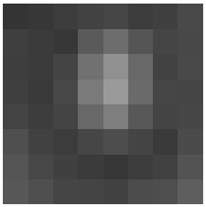Let’s for the record, say that this 8x8 image contains the following values.The range of the pixels intensities now are from 0 to 255. We will change the range from -128 to 127.

Subtracting 128 from each pixel value yields pixel value from -128 to 127. After subtracting 128 from each of the pixel value, we got the following results.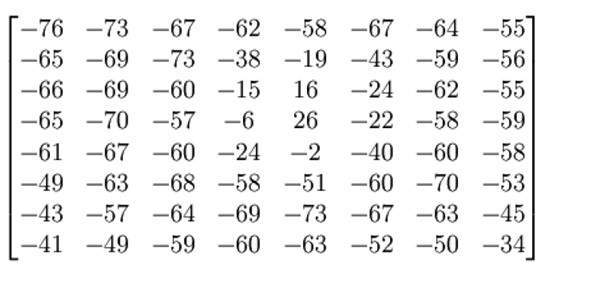Now we will compute using this formula.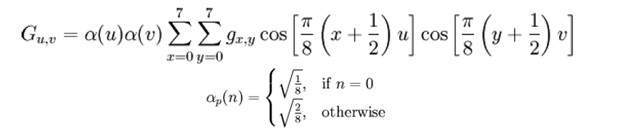The result comes from this is stored in let’s say A(j,k) matrix.

There is a standard matrix that is used for computing JPEG compression, which is given by a matrix called as Luminance matrix.

This matrix is given below### Applying the following formula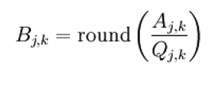We got this result after applying.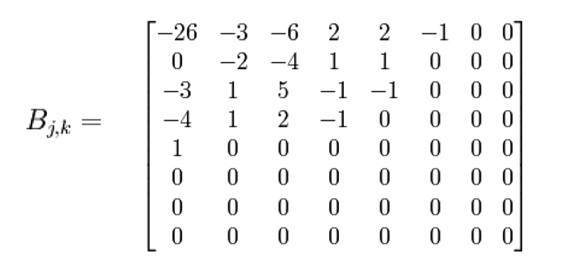Now we will perform the real trick which is done in JPEG compression which is ZIG-ZAG movement. The zig zag sequence for the above matrix is shown below. You have to perform zig zag until you find all zeroes ahead. Hence our image is now compressed.### Summarizing JPEG compression

The first step is to convert an image to Y’CbCr and just pick the Y’ channel and break into 8 x 8 blocks. Then starting from the first block, map the range from -128 to 127. After that you have to find the discrete Fourier transform of the matrix. The result of this should be quantized. The last step is to apply encoding in the zig zag manner and do it till you find all zero.

Save this one dimensional array and you are done.

Note. You have to repeat this procedure for all the block of 8 x 8.